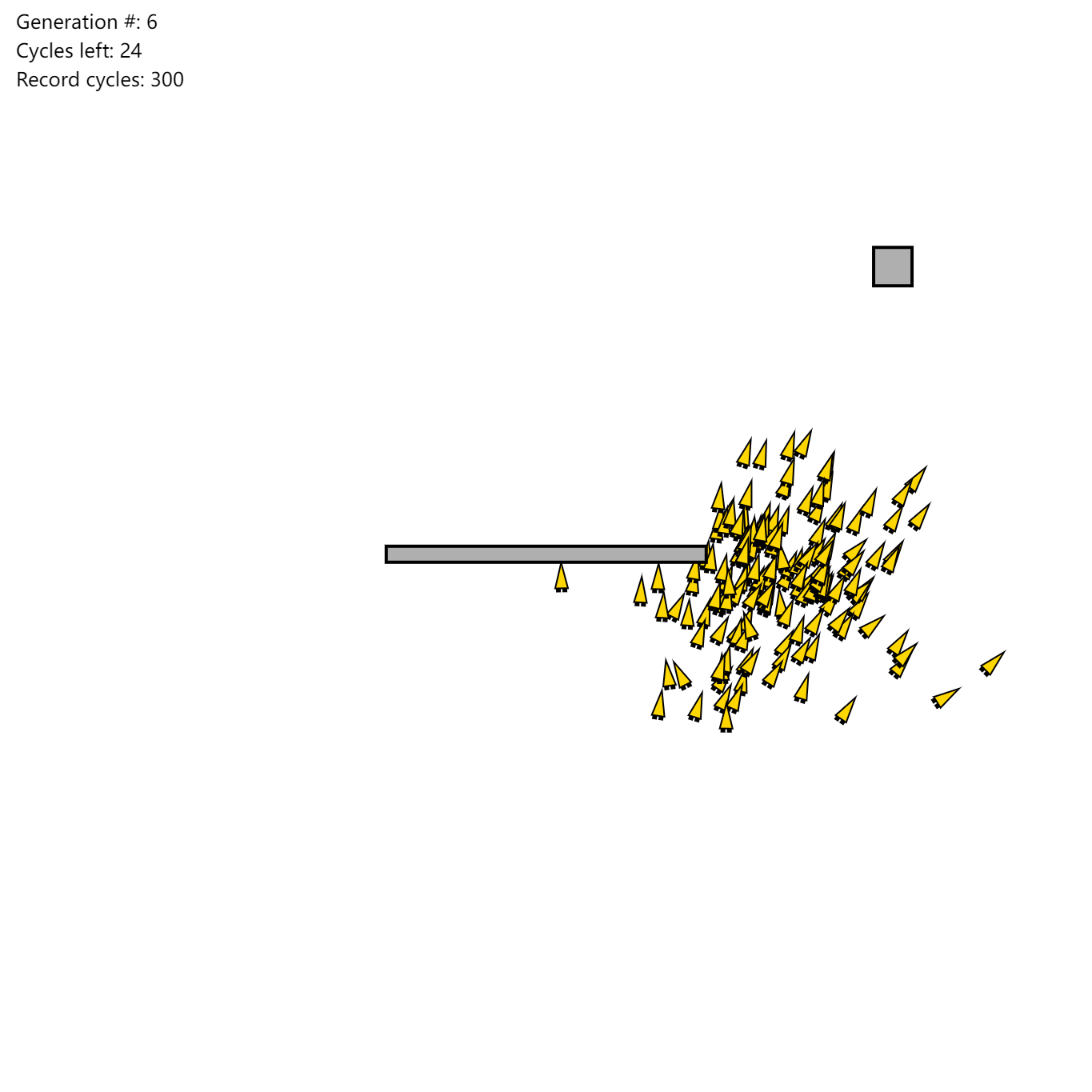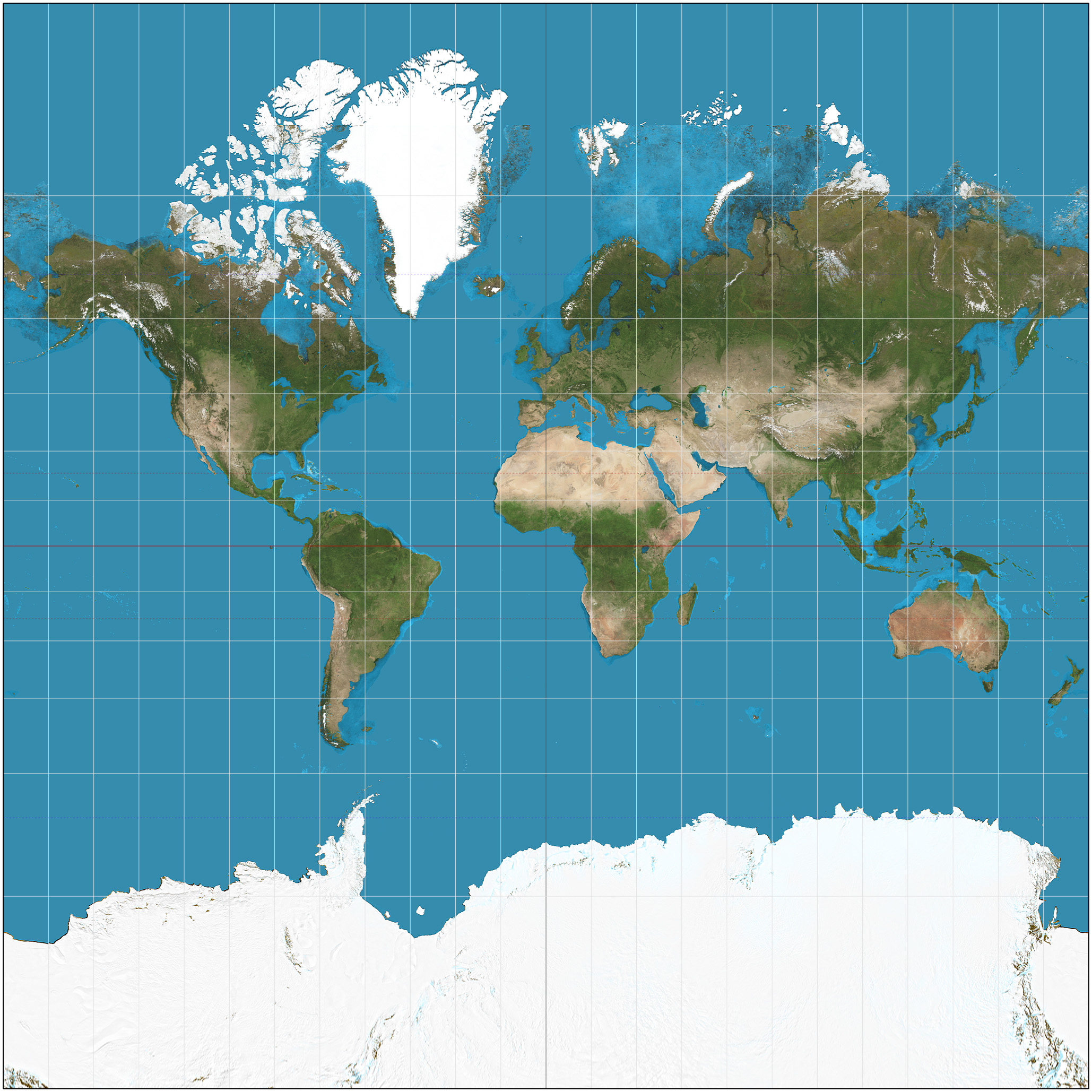By Long Luo

# 数学原理：

$h_{\theta} (x) = \theta_0 + \theta_1 x + \theta_2 x^2 + \dots + \theta_n x^n$

$J(\theta) = \frac{1}{2m} \sum_{i = 1}^{m} (h_{\theta} (x_i) - y_i)^2$

$\theta_j = \theta_j - \frac{\alpha}{m} \sum_{i = 1}^{m} (h_{\theta} (x_i) - y_i) \cdot x_j$

By Long Luo

# 原理

It’s never too late

# 举个例子By Long Luo

$\sin \angle 18^{\circ} = \frac{\sqrt {5} - 1}{4} \approx 0.3090169943$

$\sin x \approx x - \frac{x^3}{3!} + \frac{x^5}{5!} - \frac{x^7}{7!}$

$\sin \angle 18^{\circ} = \sin \frac{\pi}{10} = \frac{\pi}{10} - \frac{1}{6} (\frac{\pi}{10})^3 + \frac{1}{120} (\frac{\pi}{10})^5 - \frac{1}{5040} (\frac{\pi}{10})^7 \approx 0.30903399538$

# 泰勒公式的缺点

1. 展开过小则会导致展开精度不够，展开过大则会导致计算量过大；
2. 幂运算需要使用乘法器，存在很多重复计算；
3. 需要很多变量来存储中间值。

By Long Luo

# 如何绘制地图？By Long Luo

# 数学原理

$y_{i}\,= \,\beta _{0} + \beta _{1}x_{i} + \beta _{2}x_{i}^{2} + \cdots + \beta _{m}x_{i}^{m} + \varepsilon _{i}\ (i = 1, 2, \dots , n)$

$J(\theta ) = \sum_{i = 1}^{n} (y_i - p_m (x_i))^2$

$\frac {d(J(\theta ))}{d \theta} = 0$

$A \vec {\theta } = B$

0%